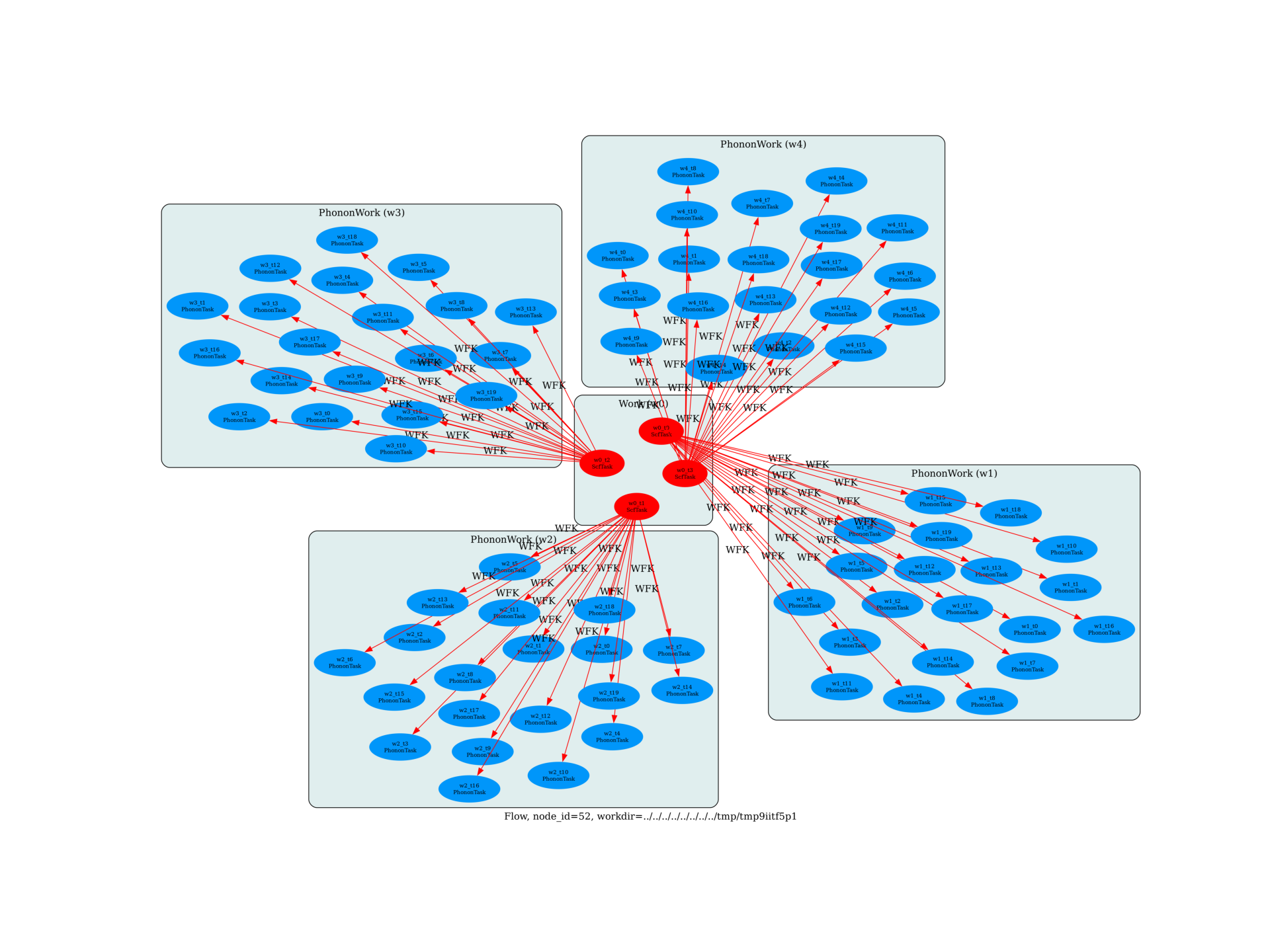Flow to analyze the convergence of phonons in metals wrt ngkpt and tsmear¶

This examples shows how to build a Flow to compute the phonon band structure in a metallic system (MgB2) with different k-point samplings and values of the electronic smearing tsmear

import os
import sys
import abipy.data as abidata
import abipy.abilab as abilab
from abipy import flowtk

def make_scf_input(structure, ngkpt, tsmear, pseudos, paral_kgb=0):
"""Build and return Ground-state input for MgB2 given ngkpt and tsmear."""

scf_inp = abilab.AbinitInput(structure, pseudos=pseudos)

# Global variables
scf_inp.set_vars(
ecut=10,
nband=8,
occopt=4,    # Marzari smearing
tsmear=tsmear,
paral_kgb=paral_kgb,
iomode=3,
)

# Dataset 1 (GS run)
scf_inp.set_kmesh(ngkpt=ngkpt, shiftk=structure.calc_shiftk())
scf_inp.set_vars(tolvrs=1e-10)

return scf_inp

def build_flow(options):
# Working directory (default is the name of the script with '.py' removed and "run_" replaced by "flow_")
if not options.workdir:
options.workdir = os.path.basename(sys.argv).replace(".py", "").replace("run_", "flow_")

structure = abidata.structure_from_ucell("MgB2")

# Get pseudos from a table.
table = abilab.PseudoTable(abidata.pseudos("12mg.pspnc", "5b.pspnc"))
pseudos = table.get_pseudos_for_structure(structure)

flow = flowtk.Flow(workdir=options.workdir)

# Build work of GS task. Each gs_task uses different (ngkpt, tsmear) values
# and represent the starting point of the phonon works.
scf_work = flowtk.Work()
ngkpt_list = [[4, 4, 4], [8, 8, 8]] #, [12, 12, 12]]
tsmear_list = [0.01, 0.02] # , 0.04]
for ngkpt in ngkpt_list:
for tsmear in tsmear_list:
scf_input = make_scf_input(structure, ngkpt, tsmear, pseudos)
flow.register_work(scf_work)

# This call uses the information reported in the GS task to
# compute all the independent atomic perturbations corresponding to a [2, 2, 2] q-mesh.
# For each GS task, construct a phonon work that will inherit (ngkpt, tsmear) from scf_task.
flow.register_work(ph_work)

return flow.allocate(use_smartio=True)

# This block generates the thumbnails in the AbiPy gallery.
# You can safely REMOVE this part if you are using this script for production runs.
__name__ = None
import tempfile
options = flowtk.build_flow_main_parser().parse_args(["-w", tempfile.mkdtemp()])
build_flow(options).graphviz_imshow()

@flowtk.flow_main
def main(options):
"""
This is our main function that will be invoked by the script.
flow_main is a decorator implementing the command line interface.
Command line args are stored in `options`.
"""
return build_flow(options)

if __name__ == "__main__":
sys.exit(main())Run the script with:

run_mgb2_phonons_nkpt_tsmear.py -s

then use:

abicomp.py ddb flow_mgb2_phonons_nkpt_tsmear/w*/outdata/*_DDB -ipy

to build a robot from the output DDB files and start the ipython shell.

then, inside the ipython shell, use:

In : %matplotlib
In : r = robot.anaget_phonon_plotters(nqsmall=0)
In : r.phbands_plotter.gridplot_with_hue("tsmear")

to compute the phonon bands with Anaddb and plot the results grouped by “tsmear”.Total running time of the script: ( 0 minutes 4.178 seconds)

Gallery generated by Sphinx-Gallery